# JP5371931B2 - Encoding device, decoding device, and methods thereof - Google Patents

## Info

Publication number
JP5371931B2
JP5371931B2 JP2010254172A JP2010254172A JP5371931B2 JP 5371931 B2 JP5371931 B2 JP 5371931B2 JP 2010254172 A JP2010254172 A JP 2010254172A JP 2010254172 A JP2010254172 A JP 2010254172A JP 5371931 B2 JP5371931 B2 JP 5371931B2
Authority
JP
Japan
Prior art keywords
spectrum
frequency spectrum
unit
low
encoding
Prior art date
Legal status (The legal status is an assumption and is not a legal conclusion. Google has not performed a legal analysis and makes no representation as to the accuracy of the status listed.)
Active
Application number
JP2010254172A
Other languages
Japanese (ja)
Other versions
JP2011043853A (en
Inventor

Original Assignee
パナソニック株式会社
Priority date (The priority date is an assumption and is not a legal conclusion. Google has not performed a legal analysis and makes no representation as to the accuracy of the date listed.)
Filing date
Publication date
Priority to JP2004145425 priority Critical
Priority to JP2004145425 priority
Priority to JP2004322953 priority
Priority to JP2004322953 priority
Priority to JP2005133729 priority
Priority to JP2005133729 priority
Priority to JP2010254172A priority patent/JP5371931B2/en
Application filed by パナソニック株式会社 filed Critical パナソニック株式会社
Publication of JP2011043853A publication Critical patent/JP2011043853A/en
Application granted granted Critical
Publication of JP5371931B2 publication Critical patent/JP5371931B2/en
Application status is Active legal-status Critical
Anticipated expiration legal-status Critical

• 238000001228 spectrum Methods 0 abstract 6
• 230000004048 modification Effects 0 abstract 3
• 238000006011 modification Methods 0 abstract 3
• 238000000034 methods Methods 0 abstract 1

## Images

•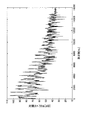•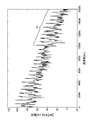••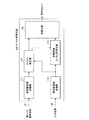•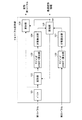•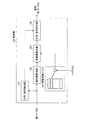•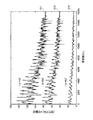•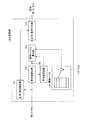•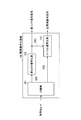•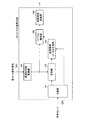•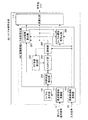•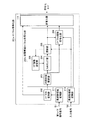••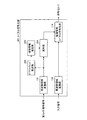•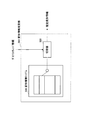•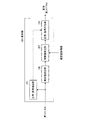•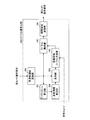•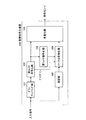•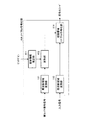•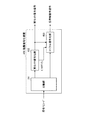•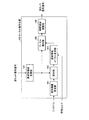•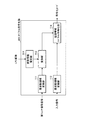•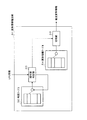•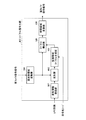•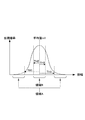•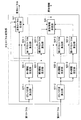•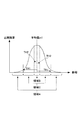•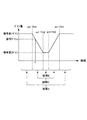•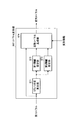## Classifications

• GPHYSICS
• G10MUSICAL INSTRUMENTS; ACOUSTICS
• G10LSPEECH ANALYSIS OR SYNTHESIS; SPEECH RECOGNITION; SPEECH OR VOICE PROCESSING; SPEECH OR AUDIO CODING OR DECODING
• G10L21/00Processing of the speech or voice signal to produce another audible or non-audible signal, e.g. visual or tactile, in order to modify its quality or its intelligibility
• G10L21/02Speech enhancement, e.g. noise reduction or echo cancellation
• G10L21/0316Speech enhancement, e.g. noise reduction or echo cancellation by changing the amplitude
• G10L21/0364Speech enhancement, e.g. noise reduction or echo cancellation by changing the amplitude for improving intelligibility
• GPHYSICS
• G10MUSICAL INSTRUMENTS; ACOUSTICS
• G10LSPEECH ANALYSIS OR SYNTHESIS; SPEECH RECOGNITION; SPEECH OR VOICE PROCESSING; SPEECH OR AUDIO CODING OR DECODING
• G10L21/00Processing of the speech or voice signal to produce another audible or non-audible signal, e.g. visual or tactile, in order to modify its quality or its intelligibility
• G10L21/02Speech enhancement, e.g. noise reduction or echo cancellation
• G10L21/038Speech enhancement, e.g. noise reduction or echo cancellation using band spreading techniques

## Abstract

There is disclosed an encoding device capable of appropriately adjusting the dynamic range of spectrum inserted according to the technique for replacing a spectrum of a certain band with a spectrum of another band. The device includes a spectrum modification unit (112) which modifies a first spectrum S1 (k) of the band 0 ‰¤ k &lt; FL in various ways to change the dynamic range so that a way of modification for obtaining an appropriate dynamic range is checked. The information concerning the modification is encoded and given to a multiplexing unit (115). By using a second spectrum S2 (k) having a valid signal band 0 ‰¤ k.

## Description

The present invention relates to an encoding device, a decoding device, and a method for encoding / decoding an audio signal, an audio signal, and the like.

Speech coding technology that compresses speech signals at a low bit rate is important for effective use of radio waves and the like in mobile communications. Furthermore, as a trend in recent years, there is an increasing expectation for improving the quality of call voice, and it is desired to realize a call service with a high presence. The sense of presence here means a sound environment (for example, BGM) surrounding the speaker, and therefore, it is desirable that signals other than voice such as audio can be encoded with high quality.

There are methods such as G726 and G729 standardized by ITU-T (International Telecommunication Union Telecommunication Standardization Sector) for voice coding for coding voice signals. These systems target narrowband signals (300 Hz to 3.4 kHz) and can encode at 8 kbit / s to 32 kbit / s. Although these schemes can be encoded at a low bit rate, the narrowband signal of interest has a narrow frequency band of up to 3.4 kHz, so its quality is steep and tends to lack realism.

In addition, ITU-T and 3GPP (The 3rd Generation Partnership Project) have standard schemes (G.722, G.722.1, AMR-WB, etc.) for encoding speech with a signal band of 50 Hz to 7 kHz. . Although these methods can encode a wideband audio signal at a bit rate of 6.6 kbit / s to 64 kbit / s, it is necessary to relatively increase the bit rate in order to encode wideband audio with high quality. . From the viewpoint of sound quality, wideband speech is higher in quality than narrowband speech, but is not sufficient for services that require a high sense of realism.

In general, when the maximum frequency of a signal is up to about 10 to 15 kHz, a sense of reality equivalent to FM radio can be obtained, and when it is up to about 20 kHz, a quality equivalent to a CD can be obtained. For a signal having such a band, audio coding represented by the Layer 3 method, the AAC method, and the like standardized by MPEG (Moving Picture Expert Group) is suitable. However, when these audio encoding methods are applied as an audio communication encoding method, it is necessary to set a high bit rate in order to encode audio with high quality. There are other problems such as an increase in coding delay.

As a method of encoding a signal with a wide frequency band with high quality at a low bit rate, the spectrum of the input signal is divided into two spectrums, a low-frequency part and a high-frequency part, and the high-frequency spectrum duplicates the low-frequency spectrum. There is a technique for reducing the overall bit rate by replacing this (substituting the high-frequency spectrum with the low-frequency spectrum) (see, for example, Patent Document 1). This technology allocates many bits to low-frequency spectrum encoding and encodes it with high quality, while high-frequency spectrum is based on duplication of low-frequency spectrum after encoding, and low bit allocation The encoding is performed at.

Further, as a technique similar to this technique, a technique for improving quality by approximating a band in which encoded bits cannot be sufficiently distributed using other predetermined partial band spectrum information (for example, Patent Document 2). Or a technique based on replicating a low-frequency spectrum of a narrow-band signal to a high-frequency spectrum in order to extend a narrow-band signal to a wide-band signal without additional information (see, for example, Patent Document 3) There is.

In any of the techniques, the spectrum of another band is duplicated in the band where the spectrum is to be replenished, and after the gain adjustment for smoothing the spectrum envelope is performed, this duplicated spectrum is inserted. .

JP-T-2001-521648 Japanese Patent Laid-Open No. 9-1553811 JP-A-9-90992

However, in the spectrum of an audio signal or audio signal, the dynamic range of the low frequency spectrum (the ratio between the maximum value and the minimum value of the absolute value (absolute amplitude) of the spectrum amplitude) is larger than the dynamic range of the high frequency spectrum. The phenomenon is often seen. FIG. 1 is a diagram for explaining this phenomenon, and shows an example of a spectrum of an audio signal. This spectrum is a logarithmic spectrum when an audio signal having a sampling frequency of 32 kHz is subjected to frequency analysis with a length of 30 ms.

As shown in this figure, the low-frequency spectrum with a frequency of 0 to 8000 Hz has strong peak characteristics (many sharp peaks exist), and the dynamic range of the spectrum in this band becomes large. On the other hand, the dynamic range of the high frequency spectrum having a frequency of 8000 to 15000 Hz is reduced. In the conventional method of replicating a low-frequency spectrum to a high-frequency spectrum for a signal having such spectral characteristics, even if the gain adjustment of the high-frequency spectrum is performed, the high-frequency spectrum is changed as shown below. Shows an unnecessary peak shape.

FIG. 2 is a diagram showing a spectrum of the entire band when a high-frequency spectrum (10000 to 16000 Hz) is obtained by duplicating the low-frequency spectrum (1000 to 7000 Hz) of the spectrum shown in FIG. 1 and adjusting the energy. It is.

When the above processing is performed, an unnecessary peak shape appears in the band R1 of 10000 Hz or higher as shown in this figure. This peak was not found in the original high frequency spectrum. Then, in the decoded signal obtained by converting this spectrum into the time domain, noise that sounds like a bell is generated, which causes a problem that the subjective quality is degraded. As described above, in the technique of substituting the spectrum of one band with the spectrum of another band, it is necessary to appropriately adjust the dynamic range of the spectrum to be inserted.

Therefore, an object of the present invention is to improve the subjective quality of a decoded signal by appropriately adjusting the dynamic range of a spectrum to be inserted in a technique for substituting (substituting) a spectrum of one band with a spectrum of another band. It is to provide an encoding device, a decoding device, and these methods capable of performing the above.

An encoding apparatus according to the present invention acquires encoding means for encoding a high frequency spectrum portion of an input signal, and a first low frequency spectrum obtained by decoding an encoded signal of a low frequency spectrum portion of the input signal. Obtaining means; estimation means for estimating information on a deformation method using dynamic range information which is a result of quantifying the dynamic range of the first low-frequency spectrum; and the first information using the estimated information. And a deformation means for generating a second low-frequency spectrum obtained by modifying the low-frequency spectrum .

The decoding device according to the present invention includes a conversion unit that generates a first low-frequency spectrum obtained by converting a signal obtained by decoding a code of a low-frequency spectrum portion included in a code generated by the encoding device into a signal in the frequency domain. a decoding means for decoding the code of the high frequency band spectrum unit included in the code generated by the encoding apparatus, modified to produce a second low frequency band spectrum obtained by modifying the amplitude of the first low frequency band spectrum And the deformation means estimates information on how to deform using dynamic range information that is a result of quantifying the dynamic range of the first low-frequency spectrum, and uses the estimated information. Then, the second low frequency spectrum is generated, and the decoding means employs a configuration in which the code of the high frequency spectrum portion is decoded using the second low frequency spectrum.

ADVANTAGE OF THE INVENTION According to this invention, in the technique which substitutes the spectrum of a certain band for the spectrum of another band, the dynamic range of the spectrum inserted can be adjusted appropriately and the subjective quality of a decoded signal can be improved.

Diagram showing an example of the spectrum of an audio signal Figure showing the spectrum of the entire band when the high-frequency spectrum is obtained by duplicating the low-frequency spectrum and adjusting the energy. FIG. 3 is a block diagram showing the main configuration of the encoding apparatus according to Embodiment 1 FIG. 2 is a block diagram showing a main configuration inside a spectrum encoding unit according to Embodiment 1 The block diagram which shows the main structures inside the spectrum transformation part which concerns on Embodiment 1. FIG. The block diagram which shows the main structures inside the deformation | transformation part which concerns on Embodiment 1. FIG. The figure which shows the example of the deformation | transformation spectrum obtained by the deformation | transformation part which concerns on Embodiment 1. FIG. The block diagram which shows the structure of another variation of the deformation | transformation part which concerns on Embodiment 1. FIG. FIG. 1 is a block diagram showing the main configuration of a hierarchical decoding apparatus according to Embodiment 1 FIG. 3 is a block diagram showing a main configuration inside a spectrum decoding unit according to the first embodiment. Block diagram for explaining a spectrum encoding unit according to Embodiment 2 The block diagram which shows the structure of another variation of the spectrum encoding part which concerns on Embodiment 2. FIG. FIG. 7 is a block diagram showing the main configuration of a spectrum decoding section according to Embodiment 2 FIG. 9 is a block diagram showing the main configuration of a spectrum encoding unit according to Embodiment 3 The figure explaining the deformation | transformation information estimation part which concerns on Embodiment 3. The block diagram which shows the main structures of the deformation | transformation part which concerns on Embodiment 3. FIG. FIG. 9 is a block diagram showing the main configuration of a spectrum decoding section according to Embodiment 3 FIG. 9 is a block diagram showing the main configuration of a hierarchical coding apparatus according to Embodiment 4 FIG. 7 is a block diagram showing the main configuration of a spectrum encoding unit according to Embodiment 4 FIG. 10 is a block diagram showing the main configuration of a hierarchical decoding apparatus according to Embodiment 4 FIG. 9 is a block diagram showing the main configuration of a spectrum decoding section according to Embodiment 4 The figure which shows the main structures of the spectrum encoding part which concerns on Embodiment 5. FIG. FIG. 9 is a block diagram showing a main configuration of a deformation information estimation unit according to Embodiment 5. The figure which shows the main structures of the spectrum decoding part which concerns on Embodiment 5. FIG. The figure for demonstrating the spectrum deformation method which concerns on Embodiment 6. FIG. The block diagram which shows the main structures inside the spectrum transformation part which concerns on Embodiment 6. FIG. The figure for demonstrating the generation method of a deformation | transformation spectrum The figure for demonstrating the generation method of a deformation | transformation spectrum FIG. 9 is a block diagram showing a main configuration inside a spectrum transformation unit according to the sixth embodiment.

Hereinafter, embodiments of the present invention will be described in detail with reference to the accompanying drawings.

(Embodiment 1)
FIG. 3 is a block diagram showing the main configuration of hierarchical coding apparatus 100 according to Embodiment 1 of the present invention. Here, a case where the encoding information has a hierarchical structure composed of a plurality of layers, that is, a case where hierarchical encoding (scalable encoding) is performed will be described as an example.

Each unit of the hierarchical encoding apparatus 100 performs the following operation in accordance with signal input.

The downsampling unit 101 generates a signal having a low sampling rate from the input signal and supplies the signal to the first layer encoding unit 102. First layer encoding section 102 encodes the signal output from downsampling section 101. The encoded code obtained by first layer encoding section 102 is given to multiplexing section 103 and also given to first layer decoding section 104. First layer decoding section 104 generates first layer decoded signal S 1 from the encoded code output from first layer encoding section 102.

On the other hand, the delay unit 105 gives a delay of a predetermined length to the input signal. This delay is for correcting a time delay generated in the downsampling unit 101, the first layer encoding unit 102, and the first layer decoding unit 104. The spectrum encoding unit 106 performs spectrum encoding of the input signal S2 delayed from the delay unit 105 by a predetermined time using the first layer decoded signal S1 generated by the first layer decoding unit 104, The generated encoded code is output to multiplexing section 103.

The multiplexing unit 103 multiplexes the encoded code obtained by the first layer encoding unit 102 and the encoded code obtained by the spectrum encoding unit 106, and outputs this as an output encoded code outside the encoding apparatus 100. Output.

FIG. 4 is a block diagram showing a main configuration inside the spectrum encoding unit 106 described above.

The spectrum encoding unit 106 mainly includes a frequency domain conversion unit 111, a spectrum modification unit 112, a frequency domain conversion unit 113, an extension band spectrum encoding unit 114, and a multiplexing unit 115.

The spectrum encoding unit 106 receives the first signal S1 having an effective signal band of 0 ≦ k <FL (k is a frequency of each subband) from the first layer decoding unit 104, and also receives an effective signal from the delay unit 105. A second signal S2 having a signal band of 0 ≦ k <FH (where FL <FH) is input. The spectrum encoding unit 106 estimates the spectrum of the band FL ≦ k <FH of the second signal S2 using the spectrum of the band 0 ≦ k <FL of the first signal S1, encodes and outputs this estimated information To do.

The frequency domain conversion unit 111 performs frequency conversion on the input first signal S1, and calculates a first spectrum S1 (k) that is a low-frequency spectrum. On the other hand, the frequency domain transform unit 113 performs frequency transform on the input second signal S2, and calculates a wide-band second spectrum S2 (k). Here, as a method of frequency conversion, discrete Fourier transform (DFT), discrete cosine transform (DCT), modified discrete cosine transform (MDCT), or the like is applied. S1 (k) is a subband spectrum of frequency k of the first spectrum, and S2 (k) is a subband spectrum of frequency k of the second spectrum.

The spectrum modification unit 112 changes the dynamic range of the first spectrum by variously modifying the first spectrum S1 (k), and examines how to modify the first spectrum S1 (k). Then, information regarding the deformation (deformation information) is encoded and supplied to the multiplexing unit 115. Details of this spectrum transformation processing will be described later. In addition, spectrum modifying section 112 outputs first spectrum S1 (k) having an appropriate dynamic range to extended band spectrum encoding section 114.

The extension band spectrum encoding unit 114 estimates the spectrum (extension band spectrum) to be included in the high band (FL ≦ k <FH) of the first spectrum S1 (k) using the second spectrum S2 (k) as a reference signal. The information related to the estimated spectrum (estimated information) is encoded and supplied to the multiplexing unit 115. Here, the estimation of the extension band spectrum is performed based on the modified first spectrum S1 ′ (k).

The multiplexing unit 115 multiplexes the encoded code of the modified information output from the spectrum modifying unit 112 and the encoded code of the estimation information related to the extended band spectrum output from the extended band spectrum encoding unit 114, and outputs the multiplexed code To do.

FIG. 5 is a block diagram showing a main configuration inside the spectrum modifying unit 112 described above.

The spectrum modification unit 112 performs a modification such that the dynamic range of the first spectrum S1 (k) is closest to the dynamic range of the high-frequency spectrum (FL ≦ k <FH) of the second spectrum S2 (k). Add to k). Then, the deformation information at this time is encoded and output.

The buffer 121 temporarily stores the input first spectrum S1 (k), and gives the first spectrum S1 (k) to the deforming unit 122 as necessary.

The deforming unit 122 generates a modified first spectrum S1 ′ (j, k) by variously modifying the first spectrum S1 (k) according to the following procedure, and supplies this to the subband energy calculating unit 123. Here, j is an index for identifying each deformation process.

The subband energy calculation unit 123 divides the frequency band of the modified first spectrum S ′ (j, k) into a plurality of subbands, and obtains subband energy (subband energy) within a predetermined range. For example, when the range for obtaining the subband energy is defined as F1L ≦ k <F1H, the subband width BWS when the bandwidth is divided into N is expressed as the following (Expression 1).
BWS = (F1H−F1L + 1) / N (Expression 1)
Therefore, the minimum frequency F1L (n) and the maximum frequency F1H (n) of the nth subband are expressed as (Equation 2) and (Equation 3), respectively.
F1L (n) = F1L + n · BWS (Formula 2)
F1H (n) = F1L + (n + 1) · BWS-1 (Formula 3)
Here, n takes a value of 0 to N-1. At this time, the subband energy P1 (j, n) is calculated as in the following (Formula 4).
Or you may obtain | require as an average value of the spectrum contained in a subband like following (Formula 5).
The subband energy P1 (j, n) obtained in this way is given to the variance calculation unit 124.

The variance calculation unit 124 calculates the variance σ1 2 (j) according to the following (Equation 6) in order to represent the degree of variation of the subband energy P1 (j, n).
Here, P1mean (j) represents the average value of the subband energy P1 (j, n), and is calculated as in the following (Expression 7).

The variance σ1 2 (j) representing the degree of variation in subband energy in the deformation information j calculated in this way is given to the search unit 125.

The subband energy calculation unit 126 and the dispersion calculation unit 127 perform the same processing as the series of processes performed by the subband energy calculation unit 123 and the dispersion calculation unit 124 on the input second spectrum S2 (k). calculating the variance .sigma. @ 2 2 representing the degree of variation of subband energy. However, the processing of the subband energy calculation unit 126 and the variance calculation unit 127 differs from the above in the following points. That is, the predetermined range for calculating the subband energy of the second spectrum S2 (k) is defined as F2L ≦ k <F2H. Here, since the dynamic range of the first spectrum needs to be close to the dynamic range of the high-frequency spectrum of the second spectrum, F2L that satisfies the condition of FL ≦ F2L <F2H is set. Also, the number of subbands for the second spectrum need not match the number N of subbands of the first spectrum. However, the number of subbands of the second spectrum is set so that the subband width of the first spectrum and the subband width of the second spectrum substantially coincide.

Searching unit 125 searches the dispersion .sigma.1 2 (j) of the dispersion .sigma.1 2 (j) and variance .sigma. @ 2 2 and most approaches when the first spectral subbands of the second spectrum subband of the first spectrum subband Determined by Specifically, the search unit 125 calculates the variance σ1 2 (j) of the first spectrum subband for all the deformation candidates 0 ≦ j <J, and calculates the calculated value and the subband of the second spectrum. The variance σ2 2 is compared to determine the value of j (optimum deformation information jopt) when the two come closest to each other, and this jopt is output to the outside of the spectrum deforming unit 112 and to the deforming unit 128.

The deforming unit 128 generates a deformed first spectrum S ′ (jopt, k) corresponding to the optimal deformation information “jopt”, and outputs it to the outside of the spectrum deforming unit 112. Note that the optimal modified information “jopt” is sent to the multiplexing unit 115, and the modified first spectrum S1 ′ (jopt, k) is sent to the extended band spectrum encoding unit 114.

FIG. 6 is a block diagram showing a main configuration inside the deformation unit 122. The internal configuration of the deformation unit 128 is basically the same as that of the deformation unit 122.

The positive / negative extraction unit 131 obtains the sign information sign (k) of each subband of the first spectrum and outputs it to the positive / negative assignment unit 134.

The absolute value calculation unit 132 calculates the absolute value of the amplitude for each subband of the first spectrum, and gives this value to the exponent value calculation unit 133.

The exponent variable table 135 records an exponent variable α (j) used for deformation of the first spectrum. A value corresponding to j among the variables included in this table is output from the exponent variable table 135. Specifically, in the exponent variable table 135, for example, exponent variable candidates consisting of four exponent variables α (j) = {1.0, 0.8, 0.6, 0.4} are recorded and specified from the search unit 125. One exponent variable α (j) is selected on the basis of the index j, and is given to the exponent value calculation unit 133.

The exponent value calculation unit 133 uses the exponent variable output from the exponent variable table 135 to calculate the exponent value of the spectrum (absolute value) output from the absolute value calculation unit 132, that is, the absolute value of the amplitude of each subband. A power raised by α (j) is calculated.

The positive / negative sign assigning unit 134 assigns the sign information sign (k) previously obtained by the positive / negative sign extracting unit 131 to the exponent value output from the exponent value calculating unit 133 and deforms it. It outputs as 1st spectrum S1 '(j, k).

Therefore, the modified first spectrum S1 ′ (j, k) output from the deforming unit 122 is expressed as the following (Expression 8).

FIG. 7 is a diagram illustrating an example of a modified spectrum obtained by the deforming unit 122 (or the deforming unit 128).

Here, the case where exponent variable α (j) = {1.0, 0.6, 0.2} is described as an example. Also, here, the spectrum S71 when α (j) = 1.0 is shifted upward by 40 dB, and the spectrum S72 when α (j) = 0.6 is shifted upward by 20 dB so that the comparison of the spectra is easy. Is displayed. From this figure, it can be seen that the dynamic range of the spectrum can be changed by the exponent variable α (j).

As described above, according to the coding apparatus (spectrum coding unit 106) according to the present embodiment, the second signal (the first signal (0 ≦ k <FL)) is used to obtain the second signal ( When the high frequency part (FL ≦ k <FH) of the second spectrum obtained from 0 ≦ k <FH) is estimated and the estimation information is encoded, the first spectrum is not used as it is, and the first spectrum is transformed into the first spectrum. The above estimation is performed after adding. At this time, information (deformation information) indicating how the image is deformed is also encoded and transmitted to the decoding side.

A specific method of deformation applied to the first spectrum is to divide the first spectrum into subbands, obtain the average absolute amplitude (subband average amplitude) of the spectrum included in each subband for each subband, and The first spectrum is modified so that the variance obtained by statistically processing the subband average amplitude is closest to the variance of the subband average amplitude obtained in the same manner from the high-frequency spectrum of the second spectrum. That is, the first spectrum is deformed so that the average amplitude of the absolute amplitude of the first spectrum and the average amplitude of the absolute amplitude of the high frequency spectrum of the second spectrum are equal. Also, the deformation information indicating this specific deformation method is encoded. Instead of the subband average amplitude, the energy of the spectrum included in each subband may be used.

More specifically, the variation of the absolute amplitude of the spectrum in the subband (shake) is controlled by raising the spectrum of the first spectrum to the power of α (0 ≦ α ≦ 1). Then, information on the used α is transmitted to the decoding side.

By adopting the above configuration, even when the dynamic range of the first spectrum and the dynamic range of the high frequency part of the second spectrum are significantly different, the dynamic range of the estimated spectrum can be appropriately adjusted, and the subjective signal of the decoded signal can be adjusted. Quality can be improved.

In the above configuration, the entire first spectrum is raised to the power of α (0 ≦ α ≦ 1), thereby uniformly limiting the amplitude of the spectrum. Thereby, a sharp (steep) peak can be blunted. In addition, for example, if the deformation is performed by simply cutting a peak above a predetermined value, the spectrum may become discontinuous and abnormal noise may be generated. However, by adopting the above configuration, the spectrum remains smooth. Occurrence of abnormal noise can be prevented.

In the present embodiment, the case where variance is used as an example of an index representing the degree of variation (amplitude) of the absolute amplitude of the spectrum has been described as an example. However, the present invention is not limited to this, and another index such as a standard deviation is applied. You may do it.

In the present embodiment, the case where an exponential function is used in the deforming unit 122 (or the deforming unit 128) in the encoding device 100 has been described as an example. However, the following method may be used.

FIG. 8 is a block diagram illustrating a configuration of another variation (deformation unit 122a) of the deformation unit. In addition, the same code | symbol is attached | subjected to the component same as the deformation | transformation part 122 (or deformation | transformation part 128), and the description is abbreviate | omitted.

In the deformation unit 122 (or the deformation unit 128), since the exponential function is used, the calculation amount tends to increase. Therefore, an increase in the amount of calculation is avoided by changing the dynamic range of the spectrum without using an exponential function.

The absolute value calculation unit 132 calculates the absolute value of each spectrum of the input first spectrum S1 (k), and outputs it to the average value calculation unit 142 and the modified spectrum calculation unit 143. The average value calculation unit 142 calculates the average value S1mean of the absolute value of the spectrum according to the following (Equation 9).

In the multiplier table 144, candidates for multipliers used in the modified spectrum calculation unit 143 are recorded. One multiplier is selected based on the index specified by the search unit 125, and is output to the modified spectrum calculation unit 143. The Here, it is assumed that four candidates of multiplier g (j) = {1.0, 0.9, 0.8, 0.7} are recorded in the multiplier table.

The modified spectrum calculation unit 143 uses the absolute value of the first spectrum output from the absolute value calculation unit 132 and the multiplier g (j) output from the multiplier table 144 to calculate the absolute value of the modified spectrum S1 ′ (k). The value is calculated according to the following (Equation 10) and output to the positive / negative sign giving unit 134.

The positive / negative sign assigning unit 134 adds the sign information sign (k) obtained by the positive / negative sign extracting unit 131 to the absolute value of the modified spectrum S1 ′ (k) output from the modified spectrum calculating unit 143. And the final deformation spectrum S1 ′ (k) expressed by the following (formula 11) is generated and output.

In the present embodiment, the case where the deformation unit includes a positive / negative sign extraction unit, an absolute value calculation unit, and a positive / negative sign assignment unit has been described as an example. However, an input spectrum is always positive. In some cases, these configurations are not necessary.

Next, the configuration of hierarchical decoding apparatus 150 that can decode the encoded code generated by hierarchical encoding apparatus 100 will be described in detail below.

FIG. 9 is a block diagram showing the main configuration of hierarchical decoding apparatus 150 according to the present embodiment.

The separation unit 151 performs separation processing on the input encoded code, and generates an encoded code S51 for the first layer decoding unit 152 and an encoded code S52 for the spectrum decoding unit 153. First layer decoding section 152 decodes the decoded signal of signal band 0 ≦ k <FL using the encoded code obtained by demultiplexing section 151, and provides this decoded signal S53 to spectrum decoding section 153. The output of the first layer decoding unit 152 is also connected to the output terminal of the decoding device 150. Thereby, when it becomes necessary to output the 1st layer decoding signal produced | generated by the 1st layer decoding part 152, it can be made to output via this output terminal.

The spectrum decoding unit 153 is provided with the encoded code S52 separated by the separation unit 151 and the first layer decoded signal S53 output from the first layer decoding unit 152. The spectrum decoding unit 153 performs spectrum decoding to be described later, generates a wideband decoded signal with a signal band 0 ≦ k <FH, and outputs this. The spectrum decoding unit 153 performs processing by regarding the first layer decoded signal S53 given from the first layer decoding unit 152 as the first signal.

FIG. 10 is a block diagram illustrating a main configuration inside the spectrum decoding unit 153 described above.

The spectrum decoding unit 153 receives the encoded code S52 and the first layer decoded signal S53 (first signal with an effective frequency band of 0 ≦ k <FL).

The separation unit 161 separates the modification information generated by the above-described spectrum modification unit 112 and the extended band spectrum coding information from the input coded code S52, and the modification information is sent to the modification unit 162. The extension band spectrum encoding information is output to extension band spectrum generation section 163.

The frequency domain transform unit 164 performs frequency transform on the input first layer decoded signal S53, which is a time domain signal, and calculates a first spectrum S1 (k). This frequency transform method uses discrete Fourier transform (DFT), discrete cosine transform (DCT), modified discrete cosine transform (MDCT), or the like.

Based on the deformation information provided from the separation unit 161, the deformation unit 162 applies deformation to the first spectrum S1 (k) provided from the frequency domain conversion unit 164 to generate a modified first spectrum S1 ′ (k). The internal configuration of the deforming unit 162 is the same as that of the encoding-side deforming unit 122 (see FIG. 6) already described, and thus the description thereof is omitted.

The extension band spectrum generation unit 163 uses the modified first spectrum S1 ′ (k) to estimate the second spectrum to be included in the extension band FL ≦ k <FH of the first spectrum S1 (k) S2 ″ ( k) and the estimated value S2 ″ (k) of the second spectrum is given to the spectrum constructing unit 165.

The spectrum constructing unit 165 combines the first spectrum S1 (k) given from the frequency domain transforming unit 164 and the estimated value S2 ″ (k) of the second spectrum given from the extended band spectrum generating unit 163 to obtain a decoded spectrum. S3 (k) is generated, and the decoded spectrum S3 (k) is expressed by the following (Equation 12).

The decoded spectrum S3 (k) is given to the time domain conversion unit 166.
After converting the decoded spectrum S3 (k) into a time domain signal, the time domain conversion unit 166 performs processing such as appropriate windowing and superposition addition as necessary to avoid discontinuities between frames. The final decoded signal is output.

Thus, according to the decoding apparatus (spectrum decoding section 153) according to the present embodiment, it is possible to decode the signal encoded by the encoding apparatus according to the present embodiment.

(Embodiment 2)
In Embodiment 2 of the present invention, the second spectrum is estimated using a pitch filter having the first spectrum as an internal state, and the characteristics of the pitch filter are encoded.

The configuration of the hierarchical encoding apparatus according to the present embodiment is the same as that of the hierarchical encoding apparatus shown in the first embodiment, and therefore spectrum encoding section 201 having a different configuration will be described using the block diagram of FIG. To do. In addition, the same code | symbol is attached | subjected to the component same as the spectrum encoding part 106 (refer FIG. 4) shown in Embodiment 1, and the description is abbreviate | omitted.

The internal state setting unit 203 sets the internal state S (k) of the filter used in the filtering unit 204 using the modified first spectrum S1 ′ (k) generated by the spectrum modifying unit 112.

The filtering unit 204 performs filtering based on the internal state S (k) of the filter set by the internal state setting unit 203 and the lag coefficient T given from the lag coefficient setting unit 206, and the second spectrum estimation value S2 "(K) is calculated. In this embodiment, a case where a filter represented by the following (formula 13) is used will be described.
Here, T represents a coefficient given from the lag coefficient setting unit 206. Here, M = 1. As shown in the following (Equation 14), the filtering process in the filtering unit 204 calculates an estimated value by multiplying and adding a coefficient β i corresponding to a spectrum lower by the frequency T in order from the lower frequency. To do.
Processing according to this equation is performed while FL ≦ k <FH. Here, S (k) represents the internal state of the filter. S (k) (where FL ≦ k <FH) calculated at this time is used as the estimated value S2 ″ (k) of the second spectrum.

Search section 205 calculates the similarity between second spectrum S2 (k) given from frequency domain transform section 113 and estimated value S2 ″ (k) of the second spectrum given from filtering section 204.

There are various definitions of this similarity, but in the present embodiment, first, filter coefficients β −1 and β 1 are regarded as 0 and defined based on the least square error (Equation 15) The similarity calculated according to is used.
In this method, the filter coefficient β i is determined after calculating the optimum lag coefficient T. Here, E represents the square error between S2 (k) and S2 ″ (k). Since the first term on the right side of the above (Equation 15) is a fixed value regardless of the lag coefficient T, ( The lag coefficient T that generates S2 ″ (k) that maximizes the second term on the right side of Equation 15) is searched. In the present embodiment, the second term on the right side of (Equation 15) is referred to as similarity.

Lag coefficient setting unit 206 sequentially outputs lag coefficient T included in predetermined search ranges TMIN to TMAX to filtering unit 204. Therefore, every time the lag coefficient T is given from the lag coefficient setting unit 206, the filtering unit 204 performs filtering after zero clearing S (k) in the range of FL ≦ k <FH, and the search unit 205 The degree of similarity is calculated every time. The search unit 205 determines a coefficient Tmax when the calculated similarity is maximum from TMIN to TMAX, and determines the coefficient Tmax as a filter coefficient calculation unit 207, a spectrum outline coding unit 208, and a multiplexing unit. 115.

The filter coefficient calculation unit 207 obtains the filter coefficient β i using the coefficient Tmax given from the search unit 205. Here, the filter coefficient β i is obtained so as to minimize the square distortion E according to the following (Equation 16).

The filter coefficient calculation unit 207 has a plurality of combinations of β i as a table in advance, determines a combination of β i that minimizes the square distortion E in (Expression 16), and multiplexes the codes. And outputs the filter coefficient β i to the spectral outline encoding unit 208.

Spectral outline coding unit 208 uses internal state S (k) given from internal state setting unit 203, lag coefficient Tmax given from search unit 205, and filter coefficient β i given from filter coefficient calculation unit 207. Then, filtering is performed to obtain an estimated value S2 ″ (k) of the second spectrum in the band FL ≦ k <FH. Then, the spectrum outline coding unit 208 calculates the estimated value S2 ″ (k) of the second spectrum and Using the second spectrum S2 (k), the spectral outline adjustment coefficient is encoded.

In the present embodiment, a case will be described in which this spectral outline information is represented by spectral power for each subband. At this time, the spectrum power of the j-th subband is expressed by the following (Equation 17).
Here, BL (j) represents the minimum frequency of the jth subband, and BH (j) represents the maximum frequency of the jth subband. The spectrum power of the subband of the second spectrum obtained in this way is regarded as the spectral outline information of the second spectrum.
Similarly, spectrum outline coding section 208 calculates subband spectral power B ″ (j) of second spectrum estimation value S2 ″ (k) according to the following (Equation 18), and changes for each subband: The quantity V (j) is calculated according to the following (Equation 19).
Next, spectrum outline coding section 208 encodes variation amount V (j) and sends the code to multiplexing section 115.

The multiplexing unit 115 includes the modification information obtained from the spectrum modification unit 112, the information about the optimum lag coefficient Tmax obtained from the search unit 205, the information about the filter coefficient obtained from the filter coefficient calculation unit 207, and the spectral outline coding. The spectral outline adjustment coefficient information obtained from the unit 208 is multiplexed and output.

As described above, according to the present embodiment, the second spectrum is estimated using the pitch filter having the first spectrum as an internal state. Therefore, only the characteristics of the pitch filter need be encoded, and the bit rate can be reduced. Is possible.

In this embodiment, the case where the frequency domain conversion unit is provided has been described. However, these are necessary components when the time domain signal is input, and when the spectrum is directly input, the frequency domain conversion is performed. Part is not necessary.

In the present embodiment, the case where M = 1 in (Expression 13) has been described as an example. However, the value of M is not limited to 1, and an integer greater than or equal to 0 can be used. .

In the present embodiment, the case where the pitch filter uses the filter function (transfer function) of (Expression 13) has been described as an example, but the pitch filter may be a primary pitch filter.

FIG. 12 is a block diagram showing a configuration of another variation (spectrum encoding unit 201a) of spectrum encoding unit 201 according to the present embodiment. In addition, the same code | symbol is attached | subjected to the component same as the spectrum encoding part 201, and the description is abbreviate | omitted.

The filter used in the filtering unit 204 is simplified as shown in the following (Equation 20).

This equation is a filter function when M = 0 and β 0 = 1 in the above (Equation 13).
The estimated value S2 ″ (k) of the second spectrum generated by this filter is obtained by sequentially copying the low-frequency spectrum of the internal state S (k) separated by T using the following (Equation 21). be able to.
In addition, as described above, the search unit 205 searches for and determines the optimum coefficient Tmax by searching for the lag coefficient T that minimizes the above (Expression 15). The coefficient Tmax obtained in this way is given to the multiplexing unit 115.

By adopting the above configuration, the filter configuration used in the filtering unit 204 is simple, so the filter coefficient calculation unit 207 is not necessary, and the second spectrum can be estimated with a small amount of calculation. That is, according to this configuration, the configuration of the encoding device is simplified, and the amount of encoding processing can be reduced.

Next, the configuration of the decoding-side spectrum decoding unit 251 capable of decoding the encoded code generated by the spectrum encoding unit 201 (or the spectrum encoding unit 201a) will be described in detail below.

FIG. 13 is a block diagram showing the main configuration of spectrum decoding section 251 according to the present embodiment. The spectrum decoding unit 251 has the same basic configuration as the spectrum decoding unit 153 (see FIG. 10) described in Embodiment 1, and the same components are denoted by the same reference numerals. The description is omitted. The difference is the internal configuration of the extended band spectrum generation unit 163a.

The internal state setting unit 252 sets the internal state S (k) of the filter used in the filtering unit 253 using the first spectrum S1 ′ (k) after deformation output from the deformation unit 162.

The filtering unit 253 obtains information related to the filter via the separation unit 161 from the encoded code generated by the encoding side spectral encoding unit 201 (201a). Specifically, in the case of the spectrum encoding unit 201, the lag coefficient Tmax and the filter coefficient β i are obtained, and in the case of the spectrum encoding unit 201a, only the lag coefficient Tmax is obtained. Then, the filtering unit 253 performs filtering based on the acquired filter information using the modified first spectrum S1 ′ (k) generated by the deforming unit 162 as the internal state S (k) of the filter, and performs the decoded spectrum S ″ ( This filtering method depends on the filter function used in the spectral-side encoding unit 201 (201a) on the encoding side, and in the case of the spectral encoding unit 201, the above-described (Equation 13) In the case of the spectrum encoding unit 201a, filtering is also performed on the decoding side according to the above (Equation 20).

The spectrum outline decoding unit 254 decodes the spectrum outline information based on the spectrum outline information given from the separation unit 161. In the present embodiment, the case where the quantized value Vq (j) of the variation amount for each subband is used will be described as an example.

The spectrum adjusting unit 255 uses the spectrum S ″ (k) obtained from the filtering unit 253 and the quantized value Vq (j) of the variation amount for each subband obtained from the spectral outline decoding unit 254 as follows (Formula 22). ) To adjust the spectrum shape of the frequency band FL ≦ k <FH of the spectrum S ″ (k) to generate the estimated value S2 ″ (k) of the second spectrum.
Here, BL (j) and BH (j) represent the minimum frequency and the maximum frequency of the jth subband, respectively. The estimated value S2 ″ (k) of the second spectrum calculated according to the above (Equation 22) is given to the spectrum configuration unit 165.

As described above in the first embodiment, the spectrum configuration unit 165 combines the first spectrum S1 (k) and the estimated value S2 ″ (k) of the second spectrum to generate a decoded spectrum S3 (k), The time domain conversion unit 166 is provided.

Thus, according to the decoding apparatus (spectrum decoding section 251) according to the present embodiment, it is possible to decode the signal encoded by the encoding apparatus according to the present embodiment.

(Embodiment 3)
FIG. 14 is a block diagram showing the main configuration of the spectrum encoding unit according to Embodiment 3 of the present invention. In FIG. 14, blocks having the same names and the same reference numerals as those in FIG. In the third embodiment, the dynamic range of the spectrum is adjusted based on information common to the encoding side and the decoding side. This eliminates the need to output an encoded code representing a dynamic range adjustment coefficient for adjusting the dynamic range of the spectrum. Since it is not necessary to output an encoded code representing a dynamic range adjustment coefficient, the bit rate can be reduced.

A spectrum encoding unit 301 in FIG. 14 includes a dynamic range calculation unit 302 and a modification information estimation unit 303 between the frequency domain conversion unit 111 and the extended band spectrum encoding unit 114 instead of the spectrum modification unit 112 in FIG. , And a deformation portion 304. The spectrum deforming unit 112 in the first embodiment changes the dynamic range of the first spectrum by variously deforming the first spectrum S1 (k), and investigates how to deform (deformation information) to have an appropriate dynamic range. The deformation information is encoded and output. On the other hand, in the third embodiment, the deformation information is estimated based on information common to the encoding side and the decoding side, and the first spectrum S1 (k) is deformed according to the estimated deformation information.

Therefore, in the third embodiment, instead of the spectrum deforming unit 112, a dynamic range calculating unit 302, a deformation information estimating unit 303, and a deforming unit 304 that deforms the first spectrum based on the estimated deformation information are provided. Since the deformation information is obtained by estimation in each of the spectrum encoding unit and the spectrum decoding unit described later, it is not necessary to output the deformation information as an encoded code from the spectrum encoding unit 301. Therefore, FIG. The multiplexing unit 115 disposed in the spectrum encoding unit 106 is not required.

The first spectrum S1 (k) is output from the frequency domain conversion unit 111 and provided to the dynamic range calculation unit 302 and the deformation unit 304. The dynamic range calculation unit 302 quantifies the dynamic range of the first spectrum S1 (k) and outputs the result as dynamic range information. As a method for quantifying the dynamic range, as in the first embodiment, the frequency band of the first spectrum is divided into a plurality of subbands, and subband energy within a predetermined range (subband energy) is obtained. A variance value of the subband energy is calculated, and this variance value is output as dynamic range information.

Next, the deformation information estimation unit 303 will be described with reference to FIG. Dynamic range information is input from the dynamic range calculation unit 302 to the deformation information estimation unit 303 and is provided to the switching unit 305. The switching unit 305 selects and outputs one estimated deformation information from the estimated deformation information candidates recorded in the deformation information table 306 based on the dynamic range information. In the deformation information table 306, a plurality of estimated deformation information candidates having values between 0 and 1 are recorded, and these candidates are determined in advance so as to correspond to the dynamic range information.

FIG. 16 is a block diagram showing a main configuration of the deformation unit 304. The blocks having the same names and the same reference numerals as those in FIG. The exponent value calculation unit 307 in the deformation unit 304 in FIG. 16 determines the absolute amplitude of the spectrum output from the absolute value calculation unit 132 according to the estimated deformation information (taken between 0 and 1) given from the deformation information estimation unit 303. The exponent value, that is, the value raised to the power of the estimated deformation information is output to the positive / negative sign giving unit 134. The positive / negative sign assigning unit 134 assigns the sign information previously obtained by the positive / negative sign extracting unit 131 to the exponent value output from the exponent value calculating unit 307 to obtain a modified first spectrum. Output.

As described above, according to the coding apparatus (spectrum coding unit 301) according to the present embodiment, the first spectrum (0 ≦ k <FL) obtained from the first signal is used to generate the second signal. When the obtained high frequency part (FL ≦ k <FH) of the second spectrum (0 ≦ k <FH) is estimated and the estimation information is encoded, the first spectrum is not used as it is, and the first spectrum is transformed. By performing the above estimation after adding, the dynamic range of the estimated spectrum can be adjusted appropriately, and the subjective quality of the decoded signal can be improved. At this time, information (deformation information) indicating how the image is deformed is for determining the deformation information based on information common to the encoding side and the decoding side (first spectrum in the third embodiment). Therefore, it is not necessary to transmit the encoded code related to the deformation information to the decoding unit, and the bit rate can be reduced.

Instead of using the deformation information table 306 to associate the dynamic range information of the first spectrum with the estimated deformation information, the deformation information estimation unit 303 uses the dynamic range information of the first spectrum as the input value and the estimated deformation. You may use the mapping function which uses information as an output value. In this case, the estimated deformation information that is the output value of the function is limited to take a value between 0 and 1.

FIG. 17 is a block diagram showing the main configuration of spectrum decoding section 353 according to Embodiment 3. In this configuration, blocks having the same names and the same reference numerals as those in FIG. Between the frequency domain conversion unit 164 and the extended band spectrum generation unit 163, a dynamic range calculation unit 361, a deformation information estimation unit 362, and a deformation unit 363 are provided. The deformation unit 162 in FIG. 10 receives the deformation information generated by the spectrum modification unit 112 on the encoding side, and transforms into the first spectrum S1 (k) given from the frequency domain transform unit 164 based on this deformation information. Apply. On the other hand, in the third embodiment, similarly to the spectrum encoding unit 301, the deformation information is estimated based on information common to the encoding side and the decoding side, and the first spectrum S1 is determined according to the estimated deformation information. The deformation of (k) is performed.

Therefore, the third embodiment includes a dynamic range calculation unit 361, a deformation information estimation unit 362, and a deformation unit 363. As with the spectrum encoding unit 301, since the deformation information is obtained by estimation inside the spectrum decoding unit, the input encoded code does not include the deformation information. The separating unit 161 arranged in the spectrum decoding unit 153 is not necessary.

The first spectrum S1 (k) is output from the frequency domain transform unit 164 and provided to the dynamic range calculation unit 361 and the deformation unit 363. Hereinafter, with respect to the operations of the dynamic range calculation unit 361, the deformation information estimation unit 362, and the deformation unit 363, the dynamic range calculation unit 302 and the deformation information in the already described encoding side spectrum encoding unit 301 (see FIG. 14). Since it is the same as that of the estimation part 303 and the deformation | transformation part 304, description is abbreviate | omitted. Note that the deformation information table in the deformation information estimation unit 362 records the same estimated deformation information candidates as the deformation information table 306 in the deformation information estimation unit 303 in the spectrum encoding unit 301.

The operations of the extended band spectrum generation unit 163, the spectrum configuration unit 165, and the time domain conversion unit 166 are the same as those described in FIG.

Thus, according to the decoding apparatus (spectrum decoding section 353) according to the present embodiment, the dynamics of the estimated spectrum can be obtained by decoding the signal encoded by the encoding apparatus according to the present embodiment. The range can be adjusted appropriately, and the subjective quality of the decoded signal can be improved.

In the present embodiment, the deformation information estimation unit 303 obtains the estimated deformation information. The estimated deformation information is applied to the spectrum encoding unit 106 shown in FIG. The estimated deformation information is provided, and the spectrum deformation unit 112 selects the deformation information in the vicinity thereof from the exponent variable table 135 based on the estimated deformation information provided from the deformation information estimation unit 303, and most of the limited deformation information. Appropriate deformation information is determined by the search unit 125. In this configuration, the encoded code of the finally selected deformation information is represented as a relative value from the reference estimated deformation information. Thus, since accurate deformation information can be encoded and transmitted to the decoding unit, an effect is obtained that the number of bits representing the deformation information can be reduced while maintaining the subjective quality of the decoded signal.

(Embodiment 4)
In Embodiment 4 of this invention, the estimated deformation | transformation information output to the deformation | transformation part in a spectrum encoding part is determined based on the pitch gain given from a 1st layer encoding part.

FIG. 18 is a block diagram showing the main configuration of hierarchical coding apparatus 400 according to the present embodiment. In FIG. 18, the blocks having the same names and the same reference numerals as those in FIG.

In hierarchical coding apparatus 400 according to the fourth embodiment, pitch gain obtained by first layer coding section 402 is provided to spectrum coding section 406. Specifically, in first layer encoding section 402, an adaptive code vector gain multiplied by an adaptive code vector output from an adaptive codebook (not shown) inherent in first layer encoding section 402 is a pitch gain. Is input to the spectrum encoding unit 406. This adaptive code vector gain is characterized by a large value when the periodicity of the input signal is strong and a small value when the periodicity of the input signal is weak.

FIG. 19 is a block diagram showing the main configuration of spectrum encoding section 406 according to Embodiment 4. In FIG. 19, blocks having the same names and reference numerals as those in FIG. The deformation information estimation unit 411 outputs estimated deformation information using the pitch gain given from the first layer encoding unit 402. The deformation information estimation unit 411 has the same configuration as the deformation information estimation unit 303 in FIG. 15 described above. However, the deformation information table designed for the pitch gain is applied. Also in this embodiment, a configuration using a mapping function may be used instead of a configuration using a deformation information table.

Thus, according to the coding apparatus (spectrum coding section 406) according to the present embodiment, the dynamic range of the estimated spectrum can be appropriately adjusted in consideration of the periodicity of the input signal, and the decoded signal The subjective quality of can be improved.

Next, the configuration of hierarchical decoding apparatus 450 capable of decoding the encoded code generated by hierarchical encoding apparatus 400 will be described below.

FIG. 20 is a block diagram showing the main configuration of hierarchical decoding apparatus 450 according to the present embodiment. In FIG. 20, the pitch gain output from first layer decoding section 452 is given to spectrum decoding section 453. In first layer decoding section 452, an adaptive code vector gain multiplied by an adaptive code vector output from an adaptive codebook (not shown) inherent in first layer decoding section 452 is output as a pitch gain, and the spectrum The data is input to the decoding unit 453.

FIG. 21 is a block diagram showing the main configuration of spectrum decoding section 453 according to the fourth embodiment. The deformation information estimation unit 461 outputs the estimated deformation information using the pitch gain given from the first layer decoding unit 452. The deformation information estimation unit 461 has the same configuration as the deformation information estimation unit 303 in FIG. 15 described above. However, the deformation information table is the same as that in the deformation information estimation unit 411, and the one designed for the pitch gain is applied. Also in this embodiment, a configuration using a mapping function may be used instead of a configuration using a deformation information table.

Thus, according to the decoding apparatus (spectrum decoding section 453) according to the present embodiment, the period of the input signal is decoded by decoding the signal encoded by the encoding apparatus according to the present embodiment. Therefore, the dynamic range of the estimated spectrum can be appropriately adjusted in consideration of the characteristics, and the subjective quality of the decoded signal can be improved.

In addition, the structure which estimates a deformation | transformation information using pitch period (Lag obtained as a result of the adaptive codebook search inherent in the 1st layer encoding part 402) with pitch gain may be sufficient. In this case, by using the pitch period, it is possible to estimate deformation information suitable for a voice with a short pitch period (for example, a female voice) and a voice with a long pitch period (for example, a male voice), and improve the estimation accuracy. Can do.

Further, in the present embodiment, the estimated deformation information is obtained in the deformation information estimation unit 411. As in the third embodiment, this estimated deformation information is stored in the spectrum encoding unit 106 shown in FIG. 4 of the first embodiment. Applying the estimated deformation information to the spectrum deformation unit 112, the spectrum deformation unit 112 selects the deformation information in the vicinity from the index variable table 135 based on the estimated deformation information provided from the deformation information estimation unit 411. The search unit 125 determines the most appropriate deformation information from the obtained deformation information. In this configuration, the encoded code of the finally selected deformation information is represented as a relative value from the reference estimated deformation information. Thus, since accurate deformation information can be encoded and transmitted to the decoding unit, an effect is obtained that the number of bits representing the deformation information can be reduced while maintaining the subjective quality of the decoded signal.

(Embodiment 5)
In Embodiment 5 of the present invention, estimated deformation information output to the deformation unit in the spectrum encoding unit is determined based on the LPC coefficient given from the first layer encoding unit.

The configuration of the hierarchical encoding apparatus in the fifth embodiment is the same as that shown in FIG. However, the parameter output from first layer encoding section 402 to spectrum encoding section 406 is not a pitch gain but an LPC coefficient.

The main configuration of spectrum encoding section 406 according to the present embodiment is as shown in FIG. The difference from FIG. 19 described above is that the parameter given to the deformation information estimation unit 511 is not a pitch gain but an LPC coefficient, and the configuration in the deformation information estimation unit 511.

FIG. 23 is a block diagram showing the main configuration of deformation information estimation section 511 according to the present embodiment. The deformation information estimation unit 511 includes a determination table 512, a similarity determination unit 513, a deformation information table 514, and a switching unit 515. Similar to the deformation information table 306 in FIG. 15, estimated deformation information candidates are recorded in the deformation information table 514. However, a candidate designed for the LPC coefficient is applied as the candidate for the estimated deformation information. The determination table 512 stores LPC coefficient candidates, and the determination table 512 and the deformation information table 514 are associated with each other. That is, when a jth LPC coefficient candidate is selected from the determination table 512, estimated deformation information suitable for the LPC coefficient candidate is stored in the jth of the deformation information table 514. The LPC coefficient has a feature that a spectrum outline (spectrum envelope) can be accurately expressed with a small number of parameters, and this spectrum outline can be associated with estimated deformation information for controlling the dynamic range. The present embodiment is configured using this feature.

The similarity determination unit 513 obtains an LPC coefficient that is most similar to the LPC coefficient given from the first layer encoding unit 402 from the determination table 512. In this similarity determination, the distance (distortion) between LPC coefficients, or the distortion of both after converting the LPC coefficient into another parameter such as an LSP (Line Spectrum Pair) coefficient, the distortion is minimized. The LPC coefficient is obtained from the determination table 512.

An index representing an LPC coefficient candidate in the determination table 512 when the distortion is minimized (that is, the highest similarity) is output from the similarity determination unit 513 and provided to the switching unit 515. The switching unit 515 selects a candidate for estimated deformation information represented by the index, and is output from the deformation information estimation unit 511.

As described above, according to the coding apparatus (spectrum coding unit 406) according to the present embodiment, the dynamic range of the estimated spectrum can be appropriately adjusted in consideration of the spectrum outline of the input signal, and decoding can be performed. The subjective quality of the signal can be improved.

Next, the configuration of a hierarchical decoding apparatus that can decode the encoded code generated by the hierarchical encoding apparatus in Embodiment 5 will be described below.

The configuration of the hierarchical decoding apparatus in the fifth embodiment is the same as that shown in FIG. However, the parameter output from first layer decoding section 452 to spectrum decoding section 453 is not a pitch gain but an LPC coefficient.

The main configuration of spectrum decoding section 453 according to the present embodiment is as shown in FIG. The difference from FIG. 21 described above is that the parameter given to the deformation information estimation unit 561 is not the pitch gain but the LPC coefficient, and the configuration in the deformation information estimation unit 561.

The configuration in the deformation information estimation unit 561 is the same as that of the deformation information estimation unit 511 in the spectrum encoding unit 406 in FIG. 22, that is, the one described in FIG. 23, and information recorded in the determination table 512 and the deformation information table 514. Is also common between the encoding side and the decoding side.

Thus, according to the decoding apparatus (spectrum decoding section 453) according to the present embodiment, the spectrum of the input signal is decoded by decoding the signal encoded by the encoding apparatus according to the present embodiment. The dynamic range of the estimated spectrum can be appropriately adjusted in consideration of the rough shape, and the subjective quality of the decoded signal can be improved.

In the present embodiment, the deformation information estimation unit 511 obtains the estimated deformation information. As in the fourth embodiment, the estimated deformation information is stored in the spectrum encoding unit 106 described in FIG. 4 of the first embodiment. Applying the estimated deformation information to the spectrum deformation unit 112, the spectrum deformation unit 112 selects the deformation information in the vicinity from the index variable table 135 based on the estimated deformation information provided from the deformation information estimation unit 511, The search unit 125 determines the most appropriate deformation information from the obtained deformation information. In this configuration, the encoded code of the finally selected deformation information is represented as a relative value from the reference estimated deformation information. Thus, since accurate deformation information can be encoded and transmitted to the decoding unit, an effect is obtained that the number of bits representing the deformation information can be reduced while maintaining the subjective quality of the decoded signal.

(Embodiment 6)
Since the basic configuration of the hierarchical coding apparatus according to Embodiment 6 of the present invention is the same as that of the hierarchical coding apparatus shown in Embodiment 1, the description thereof is omitted, and the configuration is different from that of spectrum modifying section 112. A certain spectrum modification unit 612 will be described below.

The spectrum modifying unit 612 is configured so that the dynamic range of the first spectrum S1 (k) [0 ≦ k <FL] approaches the dynamic range of the high-frequency part [FL ≦ k <FH] of the second spectrum S2 (k). The following modification is added to the first spectrum S1 (k). The spectrum modification unit 612 encodes and outputs deformation information related to the deformation.

FIG. 25 is a diagram for explaining the spectrum transformation method according to the present embodiment.

This figure shows the distribution of the amplitude of the first spectrum S1 (k). The first spectrum S1 (k) exhibits different amplitudes depending on the value of the frequency k [0 ≦ k <FL]. Therefore, when the horizontal axis represents the amplitude and the vertical axis represents the appearance probability of the amplitude, a distribution close to the normal distribution as shown in the figure centering on the average value m1 of the amplitude appears.

In the present embodiment, first, this distribution is roughly divided into a group close to the average value m1 (region B in the figure) and a group far from the average value m1 (region A in the figure). Next, representative values of the amplitudes of these two groups, specifically, the average value of the amplitude of the spectrum included in the region A and the average value of the amplitude of the spectrum included in the region B are obtained. Here, the absolute value of the amplitude when the average value m1 is converted back to zero (the average value m1 is subtracted from each value) is used as the amplitude. For example, the region A includes two regions, a region having an amplitude larger than the average value m1 and a region having an amplitude smaller than the average value m1, and the two regions can be obtained by converting the average value m1 to zero. The absolute values of the amplitudes of the spectra included in are the same. Therefore, for example, in the case of the average value in the region A, this corresponds to obtaining a representative value of the amplitude of this group by making a spectrum having a relatively large amplitude (absolute value) after conversion into one group in the first spectrum. If it is the average value of the region B, it corresponds to obtaining a representative value of the amplitude of this group by making a spectrum having a relatively small amplitude after conversion in the first spectrum as one group. Therefore, these two representative values are parameters that schematically represent the dynamic range of the first spectrum.

Next, in the present embodiment, processing similar to that performed for the first spectrum is performed on the second spectrum, and representative values corresponding to the respective groups of the second spectrum are obtained. The ratio between the representative value of the first spectrum and the representative value of the second spectrum in the region A (specifically, the ratio of the representative value of the second spectrum to the representative value of the first spectrum) and the first value in the region B A ratio between the representative value of the spectrum and the representative value of the second spectrum is obtained. Therefore, the ratio between the dynamic range of the first spectrum and the dynamic range of the second spectrum can be roughly calculated. The spectrum modification unit according to the present embodiment encodes and outputs this ratio as spectrum modification information.

FIG. 26 is a block diagram showing the main configuration inside spectrum modifying section 612.

The spectrum modification unit 612 is based on a system that calculates a representative value for each group of the first spectrum, a system that calculates a representative value for each group of the second spectrum, and a representative value calculated by these two systems. The deformation information determination unit 626 that determines the deformation information and the deformation spectrum generation unit 627 that generates a deformation spectrum based on the deformation information.

Specifically, the system for calculating the representative value of the first spectrum includes a variation degree calculation unit 621-1, a first threshold setting unit 622-1, a second threshold setting unit 623-1, and a first average spectrum. It comprises a calculation unit 624-1 and a second average spectrum calculation unit 625-1. The system for calculating the representative value of the second spectrum basically has the same configuration as the system for calculating the representative value of the first spectrum, and the same components are denoted by the same reference numerals in the figure. The difference in processing system is represented by the branch number following the symbol. The description of the same components is omitted.

The variation degree calculation unit 621-1 calculates the “variation degree” from the average value m1 of the first spectrum from the amplitude distribution of the input first spectrum S1 (k), and the first threshold setting unit 622-1. And output to the second threshold setting unit 623-1. Specifically, the “variation degree” is the standard deviation σ1 of the amplitude distribution of the first spectrum.

The first threshold value setting unit 622-1 obtains the first threshold value TH1 using the standard deviation σ1 of the first spectrum obtained by the variation degree calculating unit 621-1. Here, the first threshold value TH1 is a threshold value for specifying a spectrum having a relatively large absolute amplitude included in the region A in the first spectrum, and is a value obtained by multiplying the standard deviation σ1 by a predetermined constant a. Is used.

The operation of the second threshold setting unit 623-1 is similar to the operation of the first threshold setting unit 622-1. However, the second threshold TH2 to be obtained is a relatively absolute amplitude included in the region B of the first spectrum. And a value obtained by multiplying the standard deviation σ1 by a predetermined constant b (<a).

The first average spectrum calculation unit 624-1 obtains an average value (hereinafter referred to as a first average value) of the spectrum located outside the first threshold value TH1, that is, the spectrum included in the region A (hereinafter referred to as the first average value), and the deformation information. The data is output to the determination unit 626.

Specifically, the first average spectrum calculation unit 624-1 sets the spectrum amplitude (but the value before conversion) of each subband of the first spectrum, and sets the first threshold TH1 as the average value m1 of the first spectrum. Compared with the added value (m1 + TH1), a spectrum having an amplitude larger than this value is specified (step 1). Next, the first average spectrum calculation unit 624-1 sets the amplitude value of each subband spectrum of the first spectrum to a value obtained by subtracting the first threshold TH1 from the average value m1 of the first spectrum (m1-TH1). A spectrum having an amplitude smaller than this value is identified (step 2). The amplitude of the spectrum obtained in both step 1 and step 2 is converted to zero as the average value m1, the average value of the absolute values of the obtained converted values is obtained, and the deformation information determining unit 626 is obtained. Output to.

The second average spectrum calculation unit obtains an average value (hereinafter referred to as a second average value) of the spectrum located inside the second threshold TH2, that is, the spectrum included in the region B (hereinafter referred to as a second average value), and the deformation information determination unit 626. Output to. The specific operation is the same as that of the first average spectrum calculation unit 624-1.

The first average value and the second average value obtained by the above processing are representative values for the region A and the region B of the first spectrum.

The processing for obtaining the representative value of the second spectrum is basically the same as described above. However, since the first spectrum and the second spectrum are different spectra, a value obtained by multiplying the standard deviation σ2 of the second spectrum by a predetermined constant c is used as the third threshold TH3 according to the first threshold TH1. A value obtained by multiplying the standard deviation σ2 of the second spectrum by a predetermined constant d (<c) is used as the fourth threshold TH4 according to the second threshold TH2.

The deformation information determination unit 626 includes a first average value obtained by the first average spectrum calculation unit 624-1, a second average value obtained by the second average spectrum calculation unit 625-1, and a third average spectrum calculation unit 624-2. Using the third average value obtained in step 4 and the fourth average value obtained in the fourth average spectrum calculation unit 625-2, the deformation information is determined as follows.

That is, the deformation information determination unit 626 performs a ratio between the first average value and the third average value (hereinafter referred to as the first gain) and a ratio between the second average value and the fourth average value (hereinafter referred to as the second gain). Is called). The deformation information determination unit 626 internally includes a data table in which a plurality of encoding information candidates for deformation information is stored in advance, so that the first gain and the second gain are compared with these encoding candidates, A similar encoding candidate is selected, and an index representing the encoding candidate is output as deformation information. This index is also sent to the modified spectrum generation unit 627.

The modified spectrum generation unit 627 includes a first spectrum as an input signal, a first threshold value TH1 obtained by the first threshold value setting unit 622-1, a second threshold value TH2 obtained by the second threshold value setting unit 623-1, and modification information. Using the deformation information output from the determination unit 626, the first spectrum is deformed, and the generated deformed spectrum is output.

27 and 28 are diagrams for explaining a method of generating a modified spectrum.

The modified spectrum generation unit 627 uses the deformation information to calculate a decoded value of the ratio between the first average value and the third average value (hereinafter referred to as a decoded first gain), and a second average value and a fourth average value. A decoded value of the ratio (hereinafter referred to as a decoded second gain) is generated. These correspondences are as shown in FIG.

Next, the modified spectrum generation unit 627 identifies the spectrum belonging to the region A by comparing the amplitude value of the first spectrum with the first threshold value TH1, and multiplies these spectra by the decoded first gain. Similarly, the modified spectrum generation unit 627 identifies the spectrum belonging to the region B by comparing the amplitude value of the first spectrum with the second threshold value TH2, and multiplies these spectra by the decoded second gain.

On the other hand, as shown in FIG. 28, there is no coding information for a spectrum belonging to a region (hereinafter, region C) between the first threshold TH1 and the second threshold TH2 in the first spectrum. Therefore, the modified spectrum generation unit 627 uses a gain having an intermediate value between the decoded first gain and the decoded second gain. For example, a decoding gain y corresponding to a certain amplitude x is obtained from a characteristic curve based on the first decoding gain, the second decoding gain, the first threshold TH1, and the second threshold TH2 as shown in FIG. The gain may be multiplied by the amplitude of the first spectrum. That is, the decoding gain y is a linear interpolation value of the decoding first gain and the decoding second gain.

FIG. 29 is a block diagram showing the main configuration inside spectrum modifying section 662 used in the decoding apparatus. The spectrum deforming unit 662 corresponds to the deforming unit 162 shown in the first embodiment.

Since the basic operation is the same as that of the above-described spectrum deforming unit 612, detailed description is omitted. However, since this spectrum deforming unit 662 processes only the first spectrum, there is one processing system.

As described above, according to the present embodiment, the distribution of the amplitude of the first spectrum and the distribution of the amplitude of the second spectrum are respectively grasped and divided into a group having a relatively large absolute amplitude and a group having a relatively small absolute amplitude. Thus, the representative value of the amplitude of each group is obtained. Then, by taking the ratio of the representative value of the amplitude of each group of the first spectrum and the second spectrum, the ratio of the dynamic range between the first spectrum and the second spectrum, that is, the deformation information of the spectrum is obtained, and this is encoded. Turn into. Thereby, deformation information can be obtained without using a function with a large amount of calculation such as an exponential function.

Moreover, according to this Embodiment, a standard deviation is calculated | required from distribution of the amplitude of a 1st spectrum and a 2nd spectrum, and a 1st threshold value-a 4th threshold value are calculated | required based on this standard deviation. Therefore, since the threshold based on the actual spectrum is set, the encoding accuracy of the deformation information can be improved.

Further, according to the present embodiment, the dynamic range of the first spectrum is controlled by adjusting the gain of the first spectrum using the decoded first gain and the decoded second gain. Then, the decoded first gain and the decoded second gain are determined so that the first spectrum approaches the high frequency part of the second spectrum. Therefore, the dynamic range of the first spectrum approaches the dynamic range of the high frequency part of the second spectrum. Further, it is not necessary to use a function with a large amount of calculation such as an exponential function for calculating the decryption first gain and the decryption second gain.

In this embodiment, the case where the first decoding gain is larger than the second decoding gain has been described as an example. However, depending on the nature of the audio signal, the second decoding gain is larger than the first decoding gain. There is. That is, the dynamic range of the high frequency part of the second spectrum may be larger than the dynamic range of the first spectrum. Such a phenomenon often occurs when the input audio signal is a sound such as a frictional sound. Even in such a case, the spectrum transformation method according to the present embodiment can be applied.

Further, in the present embodiment, the case where the spectrum is divided into two groups of a group having a relatively large absolute amplitude and a group having a relatively small amplitude has been described as an example. However, in order to improve the reproducibility of the dynamic range, more You may make it divide into groups.

Further, in the present embodiment, an example has been described in which the amplitude is converted with reference to the average value, and the spectrum is divided into a relatively large group and a relatively small group based on the converted amplitude. The original amplitude value may be used as it is, and spectrum grouping may be performed based on this amplitude.

In this embodiment, the case where the standard deviation is used to calculate the degree of variation in the absolute amplitude of the spectrum has been described as an example. However, the present invention is not limited to this. For example, the variance is used as a statistical parameter similar to the standard deviation. Can be used.

In the present embodiment, the case where the average value of the absolute amplitude of the spectrum in each group is used as the representative value of the spectral amplitude of each group has been described as an example. However, the present invention is not limited to this. The median value of the absolute amplitudes may be used.

In this embodiment, the case where the amplitude value of each spectrum is used for dynamic range adjustment has been described as an example, but the energy value of the spectrum may be used instead of the amplitude value.

In addition, when obtaining a representative value corresponding to each group, for example, when the spectrum amplitude has a positive or negative sign from the beginning, such as an MDCT coefficient, it is not necessary to convert the average value to zero, What is necessary is just to obtain | require the representative value corresponding to each group using the absolute value of the amplitude of a spectrum.

The embodiments of the present invention have been described above.

The encoding device and the decoding device according to the present invention are not limited to the above embodiments, and can be implemented with various modifications.

The encoding device and the decoding device according to the present invention can also be mounted on a communication terminal device and a base station device in a mobile communication system, whereby the communication terminal device and the base station having the same operational effects as described above An apparatus can be provided.

Here, the case where the present invention is applied to a scalable coding scheme has been described as an example, but the present invention can also be applied to another coding scheme.

Further, here, a case has been described as an example where the present invention is configured with hardware, but the present invention can also be implemented with software. For example, an encoding method (decoding method) algorithm according to the present invention is described in a programming language, the program is stored in a memory, and is executed by an information processing means. A function similar to that of the decoding device can be realized.

Each functional block used in the description of each of the above embodiments is typically realized as an LSI which is an integrated circuit. These may be individually made into one chip, or may be made into one chip so as to include a part or all of them.

Although referred to as LSI here, it may be called IC, system LSI, super LSI, ultra LSI, or the like depending on the degree of integration.

Further, the method of circuit integration is not limited to LSI's, and implementation using dedicated circuitry or general purpose processors is also possible. An FPGA (Field Programmable Gate Array) that can be programmed after manufacturing the LSI or a reconfigurable processor that can reconfigure the connection or setting of circuit cells inside the LSI may be used.

Further, if integrated circuit technology comes out to replace LSI's as a result of the advancement of semiconductor technology or a derivative other technology, it is naturally also possible to carry out function block integration using this technology. There is a possibility of adaptation of biotechnology.

This description is based on Japanese Patent Application No. 2004-145425 filed on May 14, 2004, Japanese Patent Application No. 2004-322953 filed on November 5, 2004, and Japanese Patent Application No. 2005-133729 filed on April 28, 2005. All these contents are included here.

The encoding apparatus, decoding apparatus, and these methods according to the present invention can be applied to scalable encoding / decoding and the like.

111, 113 Frequency domain conversion unit 114 Spread band spectrum encoding unit 301 Spectrum encoding unit 302 Dynamic range calculation unit 303 Modification information estimation unit 304 Modification unit

## Claims (10)

1. Encoding means for encoding the high-frequency spectrum portion of the input signal;
Obtaining means for obtaining a first low frequency spectrum obtained by decoding an encoded signal of a low frequency spectrum portion of the input signal;
Estimating means for estimating information on how to deform using dynamic range information which is a result of quantifying the dynamic range of the first low-frequency spectrum;
Deformation means for generating a second low-frequency spectrum obtained by modifying the first low-frequency spectrum using the estimated information;
Equipped with,
The encoding means includes
Using the second low-frequency spectrum, estimating the high-frequency spectrum portion of the input signal, encoding the estimation result,
Encoding device.
2. The estimation means includes
Dividing the frequency band of the first low-frequency spectrum into a plurality of subbands, and using a dispersion value of energy of the subbands as the dynamic range information,
The encoding device according to claim 1.
3. The deformation means includes
Generating the second low-frequency spectrum by raising the amplitude of the first low-frequency spectrum by a predetermined value within a range from 0 to 1;
The encoding device according to claim 1.
4. Conversion means for generating a first low-frequency spectrum obtained by converting a signal obtained by decoding the code of the low-frequency spectrum portion included in the code generated by the encoding device into a signal in the frequency domain;
Decoding means for decoding the code of the high-frequency spectrum part included in the code generated by the encoding device;
Deformation means for generating a second low-frequency spectrum obtained by modifying the amplitude of the first low-frequency spectrum;
Comprising
The deformation means includes
Estimating information on how to deform using dynamic range information that is a result of quantifying the dynamic range of the first low frequency spectrum, and generating the second low frequency spectrum using the estimated information,
The decoding means includes
Decode the code of the high-frequency spectrum part to generate the estimation information of the high-frequency spectrum part, and generate the estimation spectrum of the high-frequency spectrum part by applying the estimation information to the second low-frequency spectrum To
Decryption device.
5.   A communication terminal apparatus comprising the encoding apparatus according to claim 1.
6.   A base station apparatus comprising the encoding apparatus according to claim 1.
7.   A communication terminal device comprising the decoding device according to claim 4.
8.   A base station apparatus comprising the decoding apparatus according to claim 4.
9. An encoding step for encoding the high-frequency spectrum portion of the input signal;
Obtaining a first low frequency spectrum obtained by decoding an encoded signal of a low frequency spectrum portion of the input signal;
An estimation step of estimating information on how to deform using dynamic range information that is a result of quantifying the dynamic range of the first low-frequency spectrum;
A modification step of generating a second low-frequency spectrum obtained by modifying the first low-frequency spectrum using the estimated information;
Comprising
The encoding step includes
Using the second low-frequency spectrum , estimating the high-frequency spectrum portion of the input signal , encoding the estimation result ,
Encoding method.
10. A conversion step of generating a first low-frequency spectrum obtained by converting a signal obtained by decoding a code of the low-frequency spectrum portion included in the code generated by the encoding device into a frequency-domain signal;
A decoding step of decoding a code of a high-frequency spectrum part included in the code generated by the encoding device;
A deformation step of generating a second low-frequency spectrum obtained by modifying the amplitude of the first low-frequency spectrum;
Comprising
The deformation step includes
Estimating information on how to deform using dynamic range information that is a result of quantifying the dynamic range of the first low frequency spectrum, and generating the second low frequency spectrum using the estimated information,
The decoding step includes
Decode the code of the high-frequency spectrum part to generate the estimation information of the high-frequency spectrum part, and generate the estimation spectrum of the high-frequency spectrum part by applying the estimation information to the second low-frequency spectrum To
Decryption method.
JP2010254172A 2004-05-14 2010-11-12 Encoding device, decoding device, and methods thereof Active JP5371931B2 (en)

## Priority Applications (7)

Application Number Priority Date Filing Date Title
JP2004145425 2004-05-14
JP2004145425 2004-05-14
JP2004322953 2004-11-05
JP2004322953 2004-11-05
JP2005133729 2005-04-28
JP2005133729 2005-04-28
JP2010254172A JP5371931B2 (en) 2004-05-14 2010-11-12 Encoding device, decoding device, and methods thereof

## Applications Claiming Priority (1)

Application Number Priority Date Filing Date Title
JP2010254172A JP5371931B2 (en) 2004-05-14 2010-11-12 Encoding device, decoding device, and methods thereof

## Related Child Applications (1)

Application Number Title Priority Date Filing Date
JP2006513565 Division 2005-05-13

## Publications (2)

Publication Number Publication Date
JP2011043853A JP2011043853A (en) 2011-03-03
JP5371931B2 true JP5371931B2 (en) 2013-12-18

# Family

## Family Applications (2)

Application Number Title Priority Date Filing Date
JP2006513565A Active JP4810422B2 (en) 2004-05-14 2005-05-13 Encoding device, decoding device, and methods thereof
JP2010254172A Active JP5371931B2 (en) 2004-05-14 2010-11-12 Encoding device, decoding device, and methods thereof

## Family Applications Before (1)

Application Number Title Priority Date Filing Date
JP2006513565A Active JP4810422B2 (en) 2004-05-14 2005-05-13 Encoding device, decoding device, and methods thereof

## Country Status (6)

US (1) US8417515B2 (en)
EP (3) EP2991075B1 (en)
JP (2) JP4810422B2 (en)
KR (2) KR101143724B1 (en)
BR (1) BRPI0510014B1 (en)
WO (1) WO2005111568A1 (en)

## Families Citing this family (26)

* Cited by examiner, † Cited by third party
Publication number Priority date Publication date Assignee Title
US8417515B2 (en) * 2004-05-14 2013-04-09 Panasonic Corporation Encoding device, decoding device, and method thereof
EP1742202B1 (en) * 2004-05-19 2008-05-07 Matsushita Electric Industrial Co., Ltd. Encoding device, decoding device, and method thereof
DE602007013026D1 (en) * 2006-04-27 2011-04-21 Panasonic Corp Audiocoding device, audio decoding device and method therefor
AT528750T (en) 2006-05-10 2011-10-15 Panasonic Corp Coding device and method
JP2009116245A (en) * 2007-11-09 2009-05-28 Yamaha Corp Speech enhancement device
US20090201983A1 (en) * 2008-02-07 2009-08-13 Motorola, Inc. Method and apparatus for estimating high-band energy in a bandwidth extension system
CN101971253B (en) 2008-03-14 2012-07-18 松下电器产业株式会社 Encoding device, decoding device, and method thereof
EP2320416B1 (en) * 2008-08-08 2014-03-05 Panasonic Corporation Spectral smoothing device, encoding device, decoding device, communication terminal device, base station device, and spectral smoothing method
JP5511785B2 (en) * 2009-02-26 2014-06-04 パナソニック株式会社 Encoding device, decoding device and methods thereof
JP5754899B2 (en) 2009-10-07 2015-07-29 ソニー株式会社 Decoding apparatus and method, and program
WO2011121782A1 (en) * 2010-03-31 2011-10-06 富士通株式会社 Bandwidth extension device and bandwidth extension method
JP5609737B2 (en) 2010-04-13 2014-10-22 ソニー株式会社 Signal processing apparatus and method, encoding apparatus and method, decoding apparatus and method, and program
JP5850216B2 (en) 2010-04-13 2016-02-03 ソニー株式会社 Signal processing apparatus and method, encoding apparatus and method, decoding apparatus and method, and program
ES2501840T3 (en) * 2010-05-11 2014-10-02 Telefonaktiebolaget Lm Ericsson (Publ) Procedure and provision for audio signal processing
WO2011161886A1 (en) * 2010-06-21 2011-12-29 パナソニック株式会社 Decoding device, encoding device, and methods for same
JP6075743B2 (en) * 2010-08-03 2017-02-08 ソニー株式会社 Signal processing apparatus and method, and program
US8762158B2 (en) * 2010-08-06 2014-06-24 Samsung Electronics Co., Ltd. Decoding method and decoding apparatus therefor
JP5707842B2 (en) 2010-10-15 2015-04-30 ソニー株式会社 Encoding apparatus and method, decoding apparatus and method, and program
JP6037156B2 (en) 2011-08-24 2016-11-30 ソニー株式会社 Encoding apparatus and method, and program
JP5975243B2 (en) 2011-08-24 2016-08-23 ソニー株式会社 Encoding apparatus and method, and program
WO2013051210A1 (en) * 2011-10-07 2013-04-11 パナソニック株式会社 Encoding device and encoding method
CN105324982B (en) * 2013-05-06 2018-10-12 波音频有限公司 Method and apparatus for inhibiting unwanted audio signal
WO2015041070A1 (en) 2013-09-19 2015-03-26 ソニー株式会社 Encoding device and method, decoding device and method, and program
US8879858B1 (en) * 2013-10-01 2014-11-04 Gopro, Inc. Multi-channel bit packing engine
US10410645B2 (en) 2014-03-03 2019-09-10 Samsung Electronics Co., Ltd. Method and apparatus for high frequency decoding for bandwidth extension
EP3550563A1 (en) 2014-03-31 2019-10-09 FRAUNHOFER-GESELLSCHAFT zur Förderung der angewandten Forschung e.V. Encoder, decoder, encoding method, decoding method, and program

## Family Cites Families (41)

* Cited by examiner, † Cited by third party
Publication number Priority date Publication date Assignee Title
JP3106749B2 (en) * 1992-12-10 2000-11-06 ソニー株式会社 Adaptive dynamic range encoding device
US5742734A (en) * 1994-08-10 1998-04-21 Qualcomm Incorporated Encoding rate selection in a variable rate vocoder
JP3301473B2 (en) 1995-09-27 2002-07-15 日本電信電話株式会社 Wideband audio signal restoration method
JP3283413B2 (en) 1995-11-30 2002-05-20 株式会社日立製作所 Coding decoding method, encoding apparatus and decoding apparatus
US5687191A (en) * 1995-12-06 1997-11-11 Solana Technology Development Corporation Post-compression hidden data transport
US6006108A (en) * 1996-01-31 1999-12-21 Qualcomm Incorporated Digital audio processing in a dual-mode telephone
EP0880235A1 (en) * 1996-02-08 1998-11-25 Matsushita Electric Industrial Co., Ltd. Wide band audio signal encoder, wide band audio signal decoder, wide band audio signal encoder/decoder and wide band audio signal recording medium
US6097824A (en) * 1997-06-06 2000-08-01 Audiologic, Incorporated Continuous frequency dynamic range audio compressor
SE512719C2 (en) * 1997-06-10 2000-05-02 Lars Gustaf Liljeryd A method and apparatus for reducing the data flow based on the harmonic bandwidth expansion
CA2252170A1 (en) * 1998-10-27 2000-04-27 Bruno Bessette A method and device for high quality coding of wideband speech and audio signals
JP4354561B2 (en) 1999-01-08 2009-10-28 パナソニック株式会社 Audio signal encoding apparatus and decoding apparatus
SE9903553D0 (en) * 1999-01-27 1999-10-01 Lars Liljeryd Enhancing percepptual performance of SBR and related coding methods by adaptive noise addition (ANA) and noise substitution limiting (NSL)
AUPR433901A0 (en) * 2001-04-10 2001-05-17 Lake Technology Limited High frequency signal construction method
WO2003003350A1 (en) 2001-06-28 2003-01-09 Koninklijke Philips Electronics N.V. Wideband signal transmission system
WO2003007480A1 (en) * 2001-07-13 2003-01-23 Matsushita Electric Industrial Co., Ltd. Audio signal decoding device and audio signal encoding device
JP2003108197A (en) * 2001-07-13 2003-04-11 Matsushita Electric Ind Co Ltd Audio signal decoding device and audio signal encoding device
CN1324558C (en) * 2001-11-02 2007-07-04 松下电器产业株式会社 Coding device and decoding device and audio-data distribute device
JP3926726B2 (en) * 2001-11-14 2007-06-06 松下電器産業株式会社 Encoding device and decoding device
CN100395817C (en) * 2001-11-14 2008-06-18 松下电器产业株式会社 Encoding device, decoding device and method
US7469206B2 (en) * 2001-11-29 2008-12-23 Coding Technologies Ab Methods for improving high frequency reconstruction
JP4317355B2 (en) 2001-11-30 2009-08-19 パナソニック株式会社 Encoding apparatus, encoding method, decoding apparatus, decoding method, and acoustic data distribution system
JP2003255973A (en) * 2002-02-28 2003-09-10 Nec Corp Speech band expansion system and method therefor
US6978010B1 (en) * 2002-03-21 2005-12-20 Bellsouth Intellectual Property Corp. Ambient noise cancellation for voice communication device
US20030187663A1 (en) * 2002-03-28 2003-10-02 Truman Michael Mead Broadband frequency translation for high frequency regeneration
CA2388352A1 (en) * 2002-05-31 2003-11-30 Voiceage Corporation A method and device for frequency-selective pitch enhancement of synthesized speed
US7447631B2 (en) * 2002-06-17 2008-11-04 Dolby Laboratories Licensing Corporation Audio coding system using spectral hole filling
JP3879922B2 (en) 2002-09-12 2007-02-14 ソニー株式会社 Signal processing system, signal processing apparatus and method, recording medium, and program
SE0202770D0 (en) * 2002-09-18 2002-09-18 Coding Technologies Sweden Ab Method for reduction of aliasing introduces by spectral envelope adjustment in real-valued filter bank
CA2469674C (en) * 2002-09-19 2012-04-24 Matsushita Electric Industrial Co., Ltd. Audio decoding apparatus and method
JP3854922B2 (en) 2002-10-22 2006-12-06 株式会社みずほ銀行 Transaction support method and transaction support program
KR100754439B1 (en) * 2003-01-09 2007-08-31 와이더댄 주식회사 Preprocessing of Digital Audio data for Improving Perceptual Sound Quality on a Mobile Phone
JP2004322953A (en) 2003-04-28 2004-11-18 Isono Body:Kk Thermal insulation body for vehicle, and thermal insulation panel used for the same
US7318035B2 (en) * 2003-05-08 2008-01-08 Dolby Laboratories Licensing Corporation Audio coding systems and methods using spectral component coupling and spectral component regeneration
BRPI0510303A (en) 2004-04-27 2007-10-02 Matsushita Electric Ind Co Ltd scalable coding device, scalable decoding device, and its method
US8417515B2 (en) * 2004-05-14 2013-04-09 Panasonic Corporation Encoding device, decoding device, and method thereof
US7983904B2 (en) * 2004-11-05 2011-07-19 Panasonic Corporation Scalable decoding apparatus and scalable encoding apparatus
JP2005133729A (en) 2004-11-22 2005-05-26 Takehiro Yagi Driving device using vibration shaft and movable ring
WO2006075563A1 (en) * 2005-01-11 2006-07-20 Nec Corporation Audio encoding device, audio encoding method, and audio encoding program
JP5203930B2 (en) * 2005-04-01 2013-06-05 クゥアルコム・インコーポレイテッドＱｕａｌｃｏｍｍ Ｉｎｃｏｒｐｏｒａｔｅｄ System, method and apparatus for performing high-bandwidth time axis expansion and contraction
EP1926083A4 (en) * 2005-09-30 2011-01-26 Panasonic Corp Audio encoding device and audio encoding method
AT528750T (en) * 2006-05-10 2011-10-15 Panasonic Corp Coding device and method

## Also Published As

Publication number Publication date
EP2991075A3 (en) 2016-04-06
KR101143724B1 (en) 2012-05-11
BRPI0510014B1 (en) 2019-03-26
EP2991075A2 (en) 2016-03-02
BRPI0510014A (en) 2007-09-18
EP2991075B1 (en) 2018-08-01
JP4810422B2 (en) 2011-11-09
US8417515B2 (en) 2013-04-09
EP3336843A1 (en) 2018-06-20
KR20120008537A (en) 2012-01-30
KR20070017524A (en) 2007-02-12
KR101213840B1 (en) 2012-12-20
WO2005111568A1 (en) 2005-11-24
JP2011043853A (en) 2011-03-03
US20080027733A1 (en) 2008-01-31
EP1744139A4 (en) 2011-01-19
JPWO2005111568A1 (en) 2008-03-27
EP1744139B1 (en) 2015-11-11
EP1744139A1 (en) 2007-01-17

## Similar Documents

Publication Publication Date Title
Jax et al. On artificial bandwidth extension of telephone speech
AU2008346515B2 (en) Audio encoder and decoder
RU2387024C2 (en) Coder, decoder, coding method and decoding method
EP1904999B1 (en) Frequency segmentation to obtain bands for efficient coding of digital media
JP4876574B2 (en) Signal encoding apparatus and method, signal decoding apparatus and method, program, and recording medium
CN1258171C (en) Apparatus for reinforcing information source decoder
JP5253564B2 (en) Audio coding system that uses the characteristics of the decoded signal to fit the synthesized spectral components
KR101143225B1 (en) Complex-transform channel coding with extended-band frequency coding
CN100346392C (en) Device and method for encoding, device and method for decoding
EP1905011B1 (en) Modification of codewords in dictionary used for efficient coding of digital media spectral data
JP4950210B2 (en) Audio compression
JP3881943B2 (en) Acoustic encoding apparatus and acoustic encoding method
RU2257556C2 (en) Method for quantizing amplification coefficients for linear prognosis speech encoder with code excitation
TWI441162B (en) Audio signal synthesizer, audio signal encoder, method for generating synthesis audio signal and data stream, computer readable medium and computer program
RU2389085C2 (en) Method and device for introducing low-frequency emphasis when compressing sound based on acelp/tcx
JP5255638B2 (en) Noise replenishment method and apparatus
JP4977472B2 (en) Scalable decoding device
KR101345695B1 (en) An apparatus and a method for generating bandwidth extension output data
JP3579047B2 (en) Audio decoding device, decoding method, and program
JP2006085176A (en) Band enlargement of band-limited audio signal
US8918315B2 (en) Encoding apparatus, decoding apparatus, encoding method and decoding method
KR101120911B1 (en) Audio signal decoding device and audio signal encoding device
KR101369267B1 (en) Audio encoder and bandwidth extension decoder
JP5013863B2 (en) Encoding apparatus, decoding apparatus, communication terminal apparatus, base station apparatus, encoding method, and decoding method
KR101830996B1 (en) Signal processing device and method, encoding device and method, decoding device and method, and computer readable recording medium

## Legal Events

Date Code Title Description
A977 Report on retrieval

Free format text: JAPANESE INTERMEDIATE CODE: A971007

Effective date: 20120927

A131 Notification of reasons for refusal

Free format text: JAPANESE INTERMEDIATE CODE: A131

Effective date: 20121016

A521 Written amendment

Free format text: JAPANESE INTERMEDIATE CODE: A523

Effective date: 20121213

A131 Notification of reasons for refusal

Free format text: JAPANESE INTERMEDIATE CODE: A131

Effective date: 20130611

A521 Written amendment

Free format text: JAPANESE INTERMEDIATE CODE: A523

Effective date: 20130805

TRDD Decision of grant or rejection written
A01 Written decision to grant a patent or to grant a registration (utility model)

Free format text: JAPANESE INTERMEDIATE CODE: A01

Effective date: 20130827

A61 First payment of annual fees (during grant procedure)

Free format text: JAPANESE INTERMEDIATE CODE: A61

Effective date: 20130917

R150 Certificate of patent or registration of utility model

Ref document number: 5371931

Country of ref document: JP

Free format text: JAPANESE INTERMEDIATE CODE: R150

Free format text: JAPANESE INTERMEDIATE CODE: R150

S111 Request for change of ownership or part of ownership

Free format text: JAPANESE INTERMEDIATE CODE: R313113

R350 Written notification of registration of transfer

Free format text: JAPANESE INTERMEDIATE CODE: R350